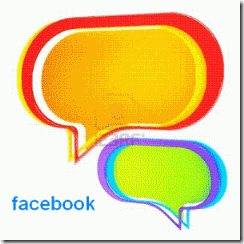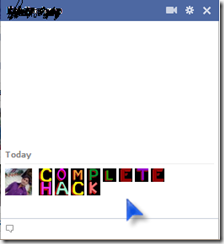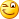## Thursday, 19 April 2012

### Colorful Messages in Facebook Chat

Make your chat more attractive by writing the message in colorful manner, This might help you to express your mood in better way.

You can send messages in colourful text but these codes will not work in Status update. This will also not work if the other person is using chat from his mobile.COMPLETE HACK  can be written as

[] [] [] [] [] [] [] []
[] [] [] [][] = A

[] = B

[] = C

[] = D

[] = E

[] = F

[] = G

[] = H

[] = I

[] = J

[] = K

[] = L

[] = M

[] = N

[] = O

[] = P

[] = Q

[] = R

[] = S

[] = T

[] = U

[] = V

[] = W

[] = X

[] = Y

[]        = Z

Have fun…..